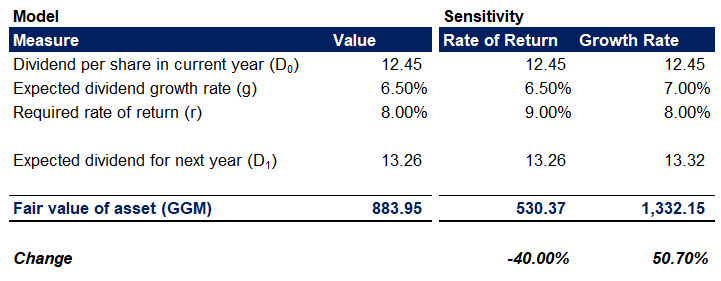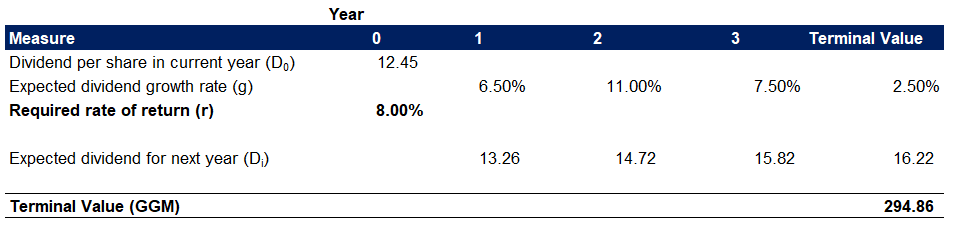## Understanding the Gordon Growth Model for Stock Valuation

Understanding the Gordon Growth Model for Stock Valuation

The Gordon Growth Model (GGM) is a method for the valuation of stocks. Investors use it to determine the relationship between value and return. The model uses the Net Present Value (NPV) of future dividends to calculate assets’ intrinsic value. It’s the most popular variation of the Dividend Discount Model (DDM).

Myron J. Gordon (alongside other academicians) published the model in 1956. A strong influence was the work of John Burr Williams and his 1938 book on the theory of investment value.

The calculation does not consider the market conditions and is only applicable to dividend-paying assets.

The model assumes there will be a stable dividend growth rate, which is not very realistic. A common way to deal with this limitation is to introduce multiple stages of the model, aiming to capture the significant rate changes over time.

## Gordon Growth Model Formula

The model consists of the following simple formula:Where:

• P0 is the price (fair value) of the asset;
• D1 is the expected dividend per share payout to common equity shareholders for next year;
• r is the required rate of return or the cost of capital;
• g is the expected dividend growth rate.

To calculate the Gordon Growth Model’s equation, we follow these steps.

First, we determine the dividend management expects to pay next year. Companies often present in their management reports the dividend growth rate they plan to achieve within the following year. If such information is not available, we can look at historical data and forecast the dividend’s development. To help with that, we can look at the expected growth of the industry or similar companies.

Second, to estimate the model’s required return rate, we can look at how the investor sees the risk and the market conditions. This is the model’s discount factor, and we can also represent it as the cost of capital, or WACC.

Last, to forecast dividend growth for the future, we look at the company’s profit projections and market expectations.

## And Get a FREE Benchmark Analysis Template

### Proving the Model

The Gordon Growth Model follows the mathematical properties of an infinite series of numbers growing at a constant rate. It uses an endless series of discounted dividend payments to calculate the fair value of a stock. We can present this with the following equation:This represents an infinite geometric sequence, which we can also define as:If we apply some calculus, which I will not get into, we can present the series as:Where the following two equations apply:andReplacing those in the geometric sequence equation, we arrive at the following:We can then multiply both the numerator and the denominator by (1+r), giving us the following:The numerator D0(1+g) represents the current year dividend increased with the expected dividend growth rate, equal to D1. If we expand the brackets in the denominator, we get the Gordon Growth Model formula:## Using the GGM in Finance

Analysts apply the Gordon Growth Model in two main ways.

They can use it to estimate the fair value of a stock and determine how viable the investment is as part of portfolio analysis. If the model’s result is above the current market price, the asset is undervalued, and it’s worth buying it. And if the modeled value is below the price, this means the asset is overvalued on the market, and it’s worth selling it. Another way to employ GGM is to compare investment opportunities and identify the better ones that would yield higher profitability.

Another way analysts may use the model is in reverse to estimate the discount factor. As this represents the cost of capital, if we know the fair value of a stock, the expected dividend, and its growth rate, we can use the equation to estimate the Weighted Average Cost of Capital (WACC) for the company.

It is important to note that we can also apply the model to scenarios with an expected negative growth rate for the dividend payouts. If it’s below zero, then the denominator (r – g) will increase, and the method will yield a lower fair value amount.

If the growth rate is equal to the required return rate, the model approaches infinity and is not viable from a mathematical perspective.

Professional valuation practices rarely use the model in its original form. Most analysts use a multistage model version, which may have various stages like ‘high growth,’ ‘stable,’ and others. Many companies also have their ‘proprietary’ models, that are often kept as trade secrets and include additional assumptions and parameters

## Assumptions

The Gordon Growth Model is only viable if it meets certain conditions.

• The company has a stable business model, and there will be no significant changes in its operations;
• All free cash flow that remains at the end of the year is paid out as dividends at regular intervals;
• We expect dividends to grow at a stable rate;
• We also expect the business to grow at the same stable rate;
• Growth rate (g) has to be less than the required rate of return (r);

The method gives a clear relation between value and return. It’s a useful model to analyze stock values, but it has some benefits and drawbacks as other predictive models in finance.

If we calculate the model under the right conditions, meaning all model assumptions are met, we get the following advantages:

• The method is easy to use – it only takes three inputs, and it’s simple to calculate. This makes it much more straightforward compared to other valuation techniques;
• Inputs are easy to obtain from management reports or the corporate strategy of the company, often shared on their website;
• The model is beneficial when benchmarking dividend-paying stocks.

On the other hand, the GGM has some disadvantages, which may hinder its performance and lead to ineffective valuation:

• The dividend growth rate has to be constant or at a limited number of stages (for multistage models). Such stable rates are applicable mostly to mature stable companies and not very common to new and developing businesses and industries, where there’s still much inherent volatility. In reality, companies can experience fluctuations in the growth rate due to business cycles and unforeseen successes and setbacks;
• The model assumes the company will exist forever and grow forever, paying dividends at a continually increasing rate.
• The rate of return (discount factor) has to be larger than the growth rate. Otherwise, the model yields a negative value, which is not usable;
• The method ignores external factors, like market conditions, that may impact the value of the stock significantly;
• GGM only works for dividend-paying companies, which limits the cases where we can apply the model. To increase growth, newer companies tend to reinvest profits instead of paying out dividends;
• Mature companies may often strive to pay more dividends to manifest their stability and strength in front of shareholders.

The model’s most significant flaws are the assumption for a constant growth rate and the high sensitivity towards small changes in growth rate and discount factor.

# Example Gordon Growth Model in Excel

To solidify the gained knowledge, let us set up a sample Gordon Growth Model in Excel.

### Classic Gordon Growth Model

We start with a simple single-stage model. We have a current year dividend payout at €12.45. The company’s expected dividend growth rate in perpetuity is 6.5%, and the required rate of return (the discount factor) is 8%.

We first need to calculate the expected dividend payout in the next year (D1), and then we can apply the GGM formula to arrive at the current fair value of the stock, or €883.95.This is the most straightforward implementation of the method. However, let’s also illustrate how sensitive the model is to the inherent rate assumptions. You can see that by increasing the required rate of return (r) by 1%, we get a 40% decrease in the stock’s fair value. Alternatively, if we increase the expected dividend growth rate by only 0.50%, we get a 51% increase in fair value. This illustrates the importance of setting up proper and realistic assumptions for our model. The model is an effective valuation method, but its sensitivity towards the two rates is its biggest drawback.Additionally, let’s illustrate the two edge cases that we discussed below. If the expected growth rate is equal to the required return rate (g = r), it’s impossible to calculate the GGM equation in Excel. Mathematically, division over zero approaches infinity. In such a case, the method is not a viable valuation technique, as it suggests the stock has infinite value.

We can also illustrate a case where we expect a negative dividend growth rate (g). Having such will increase the denominator and result in a much lower value of the stock’s fair value.### Multistage Gordon Growth Model

Let us now illustrate a more realistic scenario, where we have different stages of our expected dividend growth rate. We have refined our rate forecast and have the following inputs for our first three years and a terminal value from thereon. After the third year, we expect a stable dividend growth rate of 2.5%. The required rate of return (the discount factor) and the dividend payout remain the same in the current year.First, let’s calculate the expected dividend for each of the next years. Here’s is where we would apply the GGM equation to calculate the terminal value at the end of the third year.We can now discount the expected dividends for the next three years and the terminal value. Adding those up, we get the present fair value of the stock at €271.52.Whenever we build models with high sensitivity to assumptions, it is a great idea to add a sensitivity table to our model. This table shows how changes in the expected perpetual dividend growth rate after the third year (g) and the required return rate (r) will impact the fair value calculation.## Conclusion

The Gordon Growth Model is the most popular variant of the Dividend Discount Model. It is an effective way to analyze investment opportunities and compare stocks.

When we work with the model, it is crucial to keep close attention to our assumptions’ volatility, as we illustrated how sensitive the GGM is to the dividend growth rate and the required rate of return.## FCCA, FMVA

Hi! I am a finance professional with 10+ years of experience in audit, controlling, reporting, financial analysis and modeling. I am excited to delve deep into specifics of various industries, where I can identify the best solutions for clients I work with.

In my spare time, I am into skiing, hiking and running. I am also active on Instagram and YouTube, where I try different ways to express my creative side.